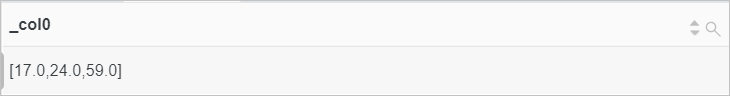This topic describes the syntax of approximate functions. This topic also provides examples on how to use the functions.

Function Syntax Description
approx_distinct function approx_distinct(x) Estimates the number of unique values in a specified field. The standard error is 2.3%.
numeric_histogram function numeric_histogram(bucket,x) Computes the approximate histogram of a specified field based on the number of histogram columns specified by the bucket parameter. The returned result is of the JSON type.
numeric_histogram_u function numeric_histogram_u(bucket,x) Computes the approximate histogram of a specified field based on the number of histogram columns specified by the bucket parameter. A table that includes multiple rows and columns is returned.
approx_percentile function approx_percentile(x,percentage) Lists the values of a specified field in ascending order and returns the value that is approximately at the percentage position.
approx_percentile(x,array[percentage01,percentage02...]) Lists the values of a specified field in ascending order and returns the values that are approximately at the percentage01 and percentage02 positions.

## approx_distinct function

The approx_distinct function is used to estimate the number of unique values in a specified field. The standard error is 2.3%.

### Syntax

``approx_distinct(x)``

### Parameters

Parameter Description
x The value of this parameter is of the arbitrary data type.

The bigint type.

### Examples

Use the count function to calculate the number of page views (PVs), and then use the approx_distinct function to estimate the unique values of the client_ip field as the number of unique visitors (UVs).

• Query statement
``* |SELECT count(*) AS PV, approx_distinct(client_ip) AS UV``
• Query and analysis result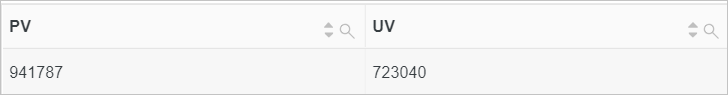## numeric_histogram function

The numeric_histogram function is used to compute the approximate histogram of a specified field based on the number of histogram columns specified by the bucket parameter. The returned result is of the JSON type.

### Syntax

``numeric_histogram(bucket,x)``

### Parameters

Parameter Description
bucket The number of columns in the histogram. The value of this parameter is of the bigint type.
x The value of this parameter is of the double type.

The JSON type.

### Examples

Compute the approximate histogram of the request duration for the POST method.

• Query statement
``request_method:POST | SELECT numeric_histogram(10,request_time)``
• Query and analysis result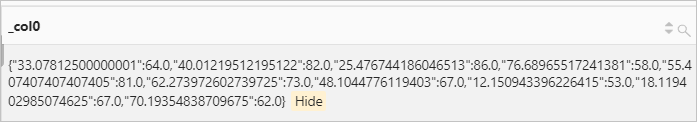## numeric_histogram_u function

The numeric_histogram_u function is used to compute the approximate histogram of a specified field based on the number of histogram columns specified by the bucket parameter. A table that includes multiple rows and columns is returned.

### Syntax

``numeric_histogram_u(bucket,x)``

### Parameters

Parameter Description
bucket The number of columns in the histogram. The value of this parameter is of the bigint type.
x The value of this parameter is of the double type.

The double type.

### Examples

Compute the approximate histogram of the request duration for the POST method.

• Query statement
``request_method:POST | select numeric_histogram_u(10,request_time)``
• Query and analysis result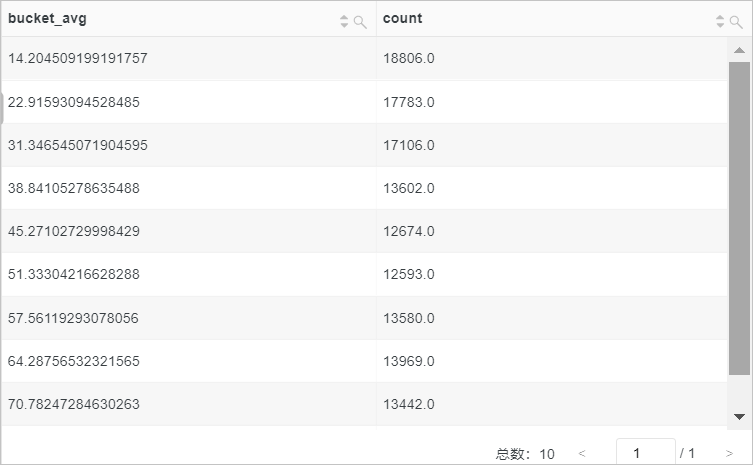## approx_percentile function

The approx_percentile function is used to list the values of a specified field in ascending order, and then return the value that is approximately at the percentage position.

### Syntax

• To return the value that is approximately at the percentage position, use the following syntax. The returned result is of the double type.
``approx_percentile(x,percentage)``
• To return the values that are approximately at the percentage01 and percentage02 positions, use the following syntax. The returned result is of the array type.
``approx_percentile(x,array[percentage01,percentage02...])``

### Parameters

Parameter Description
x The value of this parameter is of the double type.
percentage The percentage value. Value range: [0,1].

### Return value type

The double type or array type.

### Examples

• Example 1: Sort the values of the request_time column in ascending order, and then return the value that is approximately at the 50% position in the request_time field.
• Query statement
``*| select approx_percentile(request_time,0.5)``
• Query and analysis result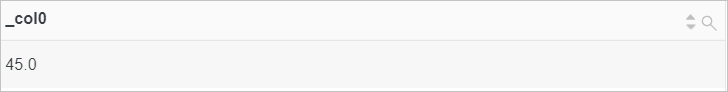• Example 2: Sort the values of the request_time column in ascending order, and then return the values that are approximately at the 10%, 20%, and 70% positions in the request_time field.
• Query statement
``*| select approx_percentile(request_time,array[0.1,0.2,0.7])``
• Query and analysis result